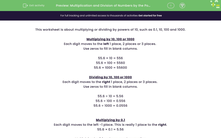# Multiply and Divide Numbers by Powers of 10, Including 0.1

In this worksheet, students will multiply or divide numbers by powers of 10 (0.1 to 1000).Key stage:  KS 3

Curriculum topic:   Number

Curriculum subtopic:   Use Four Operations for All Numbers

Difficulty level:#### Worksheet Overview

This activity is about multiplying or dividing by powers of 10, such as 0.1, 10, 100 and 1000.

Multiplying by 10, 100 or 1000

Each digit moves to the left 1 place, 2 places or 3 places.

Use zeros to fill in blank columns.

55.6 × 10 = 556

55.6 × 100 = 5560

55.6 × 1000 = 55600

Dividing by 10, 100 or 1000

Each digit moves to the right 1 place, 2 places or 3 places.

Use zeros to fill in blank columns.

55.6 ÷ 10 = 5.56

55.6 ÷ 100 = 0.556

55.6 ÷ 1000 = 0.0556

Multiplying by 0.1

0.1 means one tenth, so this is the same as dividing by 10.

So, each digit moves 1 place to the right.

55.6 × 0.1 = 5.56

Multiplying by 0.1 makes a number smaller.

Dividing by 0.1

0.1 means one tenth, so this is the same as multiplying by 10.

So, each digit moves 1 place to the left.

55.6 ÷ 0.1 = 556

Dividing by 0.1 makes a number bigger.

Note that, although large numbers would normally include commas to separate the thousands, in this activity we have omitted the commas in case they were confused for the decimal point!

The best way to understand this is to practise by doing some questions - so what are we waiting for?!### What is EdPlace?

We're your National Curriculum aligned online education content provider helping each child succeed in English, maths and science from year 1 to GCSE. With an EdPlace account you’ll be able to track and measure progress, helping each child achieve their best. We build confidence and attainment by personalising each child’s learning at a level that suits them.

Get started